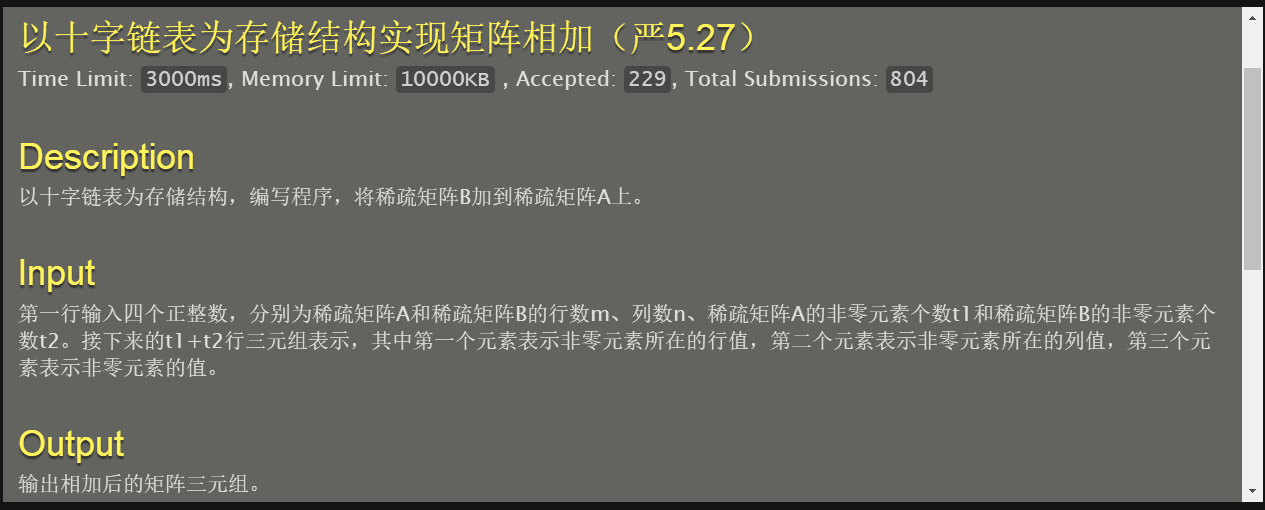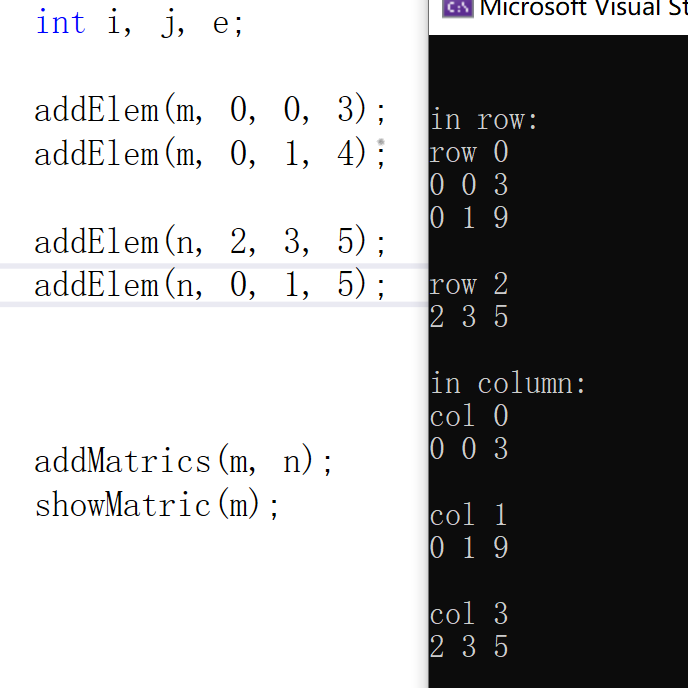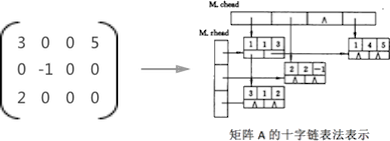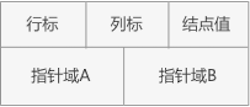• 十字链表加法

2018-04-26 15:02:13
计算十字链表的加法程序，采用C++编写。十字链表主要用于稀疏矩阵的存储，是压缩存储的一大利器十字链表
•十字链表
• 创建十字链表并不是非常难。我借鉴了这位（https://blog.csdn.net/zhuyi2654715/article/details/6729783）的写法，写的很清楚，但是不能实现同一处连续插入两次的操作。 最难的还是加法。我们假设把B加到A上面，...

题目复制粘贴感谢https://blog.csdn.net/npuyan/article/details/80464459?spm=1001.2014.3001.5501
这位代码写的很好

细节

创建十字链表并不是非常难。我借鉴了这位（https://blog.csdn.net/zhuyi2654715/article/details/6729783）的写法，写的很清楚，但是不能实现同一处连续插入两次的操作。
最难的还是加法。我们假设把B加到A上面，那么，就存在添加新结点，不加不减（做加法未得到0），删除结点（做加法得到0）三种情况。对于删除结点呢，我们先从水平方向遍历，确定要删的结点——但是还不能删除（否则垂直方向会导致野指针出现），所以只能先标记，等之后垂直方向遍历时再删掉。
更糟糕的是，由于我们创建十字链表为了节省空间，没有采用头结点而是头指针，所以对于某一行或者某一列只有一个结点的情况，还要单独处理（自闭），这时候，有一个前驱指针就显得极其重要了。这是我大意没有闪的一处

代码

#include <stdio.h>
#include <stdlib.h>
using namespace std;
typedef struct node
{
int i,j,v;
struct node *right,*down;
}OLNode,*OList;
typedef struct
{
int mu,nu,tu;
}CrossList;

void Create_CrossList(CrossList *L,int m,int n,int t)
{
{
printf("十字链表指针数组分配空间失败\n");
exit(-1);
}
{
printf("十字链表指针数组分配空间失败\n");
exit(-1);
}
L->mu=m,L->nu=n,L->tu=t;
int i;
for(i=1;i<=m;i++)
{
}
for(i=1;i<=n;i++)///初始化指针数组
{
}
for(i=1;i<=t;i++)
{
int r,c,v;
scanf("%d%d%d",&r,&c,&v);
OList node=(OList)malloc(sizeof(OLNode));
if(!node)
{
printf("结点内存分配失败\n");
exit(-1);
}
node->i=r,node->j=c,node->v=v;
{
}
else
{
OList temp;
///寻找插入位置
node->right=temp->right;
temp->right=node;
}
{
}
else
{
OList temp;
///寻找插入位置
node->down=temp->down;
temp->down=node;
}
}
}

void Insert(CrossList *A,OList node)
{
int r=node->i,c=node->j,v=node->v;
{
A->tu++;
}
else
{
while(temp&&temp->j<c)///找到插入位置。保留前驱pre非常有用。
{
pre=temp;
temp=temp->right;
}
if(!temp)///因为第r行至少有一个结点，则temp为NULL说明pre->j<c，需要插入结点
{
pre->right=node;
A->tu++;
}
else if(temp->j==c)///等于要分情况:有一个结点和有多个结点
{
temp->v+=v;
if(!temp->v)
{
del=1;
A->tu--;
if(pre==temp)///我们只有头指针，没有头结点，所以pre==temp这里要特殊处理
{
}
else
{
pre->right=temp->right;///有多个结点
}
}
}
else if(temp->j>c)///这一段不能省去。
{
A->tu++;
node->right=temp,pre->right=node;
}
}
{
}
else
{
while(temp&&temp->i<r)///找到插入位置。保留前驱pre非常有用。
{
pre=temp;
temp=temp->down;
}
{
node->down=temp;
pre->down=node;
}
else if(del==1)///需要删除
{
pre->down=temp->down;
free(temp),free(node);
}
}
}
{
OList now;
int k;
for(k=1;k<=B->mu;k++)
{
while(now)
{
OList temp=(OList)malloc(sizeof(OLNode));
temp->i=now->i,temp->j=now->j,temp->v=now->v;
temp->down=now->down,temp->right=now->right;///做一份拷贝，因为插入时涉及down和right域的改变
Insert(A,temp);
now=now->right;
}
}
}
void print_result(CrossList *A)
{
int k;
for(k=1;k<=A->mu;k++)
{
while(temp)
{
printf("%d %d %d\n",temp->i,temp->j,temp->v);
temp=temp->right;
}
}
}
int main()
{
CrossList *A=(CrossList*)malloc(sizeof(CrossList));
CrossList *B=(CrossList*)malloc(sizeof(CrossList));
int m,n,t1,t2;
scanf("%d%d%d%d",&m,&n,&t1,&t2);
Create_CrossList(A,m,n,t1);///创建十字链表
Create_CrossList(B,m,n,t2);
print_result(A);///输出结果
free(A),free(B);
return 0;
}
/*
3 4 3 2
1 1 1
1 3 1
2 2 2
1 2 1
2 2 3
*/
/*
3 4 3 2
1 1 4
2 2 3
3 3 2
1 1 -4
2 2 -3
*/

展开全文• 稀疏矩阵加法十字链表）问题描述输入输出输入输出样例代码 问题描述 若将稀疏矩阵 \mathbf{A}A 的非零元素以行序为主序的顺序存于一维数组 VV 中，并用二维数组 BB 表示 AA 中的相应元素是否为零元素（以0和1分别...

问题描述

若将稀疏矩阵 \mathbf{A}A 的非零元素以行序为主序的顺序存于一维数组 VV 中，并用二维数组 BB 表示 AA 中的相应元素是否为零元素（以0和1分别表示零元素和非零元素）。例如，可以使用一个数组和一个01矩阵表示试写一算法，实现在上述表示法中实现矩阵相加的运算。并分析你的算法时间复杂度（在代码注释中给出）。

输入输出输入：

2 2
1 1
1 0
0 1
-2 -1
1 0
0 1

输出：

-1
1 0
0 0

代码

#include <stdio.h>
#include <stdlib.h>
//十字链表的结点
typedef struct olnode{
//line
int i;
//row
int j;
//elemtype
int e;
//right
struct olnode *right;
//down
struct olnode *down;
}ol;

//存储行和列的头
typedef struct {
//line
int mu;
//row
int nu;
//element
int tu;
}crosslist;

//申请内存
ol *lalloc(void){
return (ol *)malloc(sizeof(ol));
}

int main(){
int m,n;
//读入行数和列数
scanf("%d %d\n",&m,&n);

//获取输入并返回数组长度
int getinput(int *p);
//获取输入的矩阵
crosslist getarray(crosslist p,int v[]);
//初始化头链表
//相加
void addnode(crosslist p,int ia,int ja,int k,int v[]);
//打印v数组
void printv(crosslist a);
//打印01矩阵
void printm(crosslist a);

//根据行数和列数
int va[m*n];
int vb[m*n];
int vc[m*n];

//存储行和列的头
//读入va
int i,j;
//读入数组a
//读入vb
//读入数组b
//相加

//输出

return 0;
}

//读入数组va，p为数组名
int getinput(int *p){
int c;
int i=0;
//符号位
int sig=1;
c=getchar();
if(c=='\n'){
c=getchar();
}
while(c!='\n'){
sig=1;
if(c==' '){
i++;
c=getchar();
}
if(c=='-'){
sig=-1;
c=getchar();
}
p[i]=c-'0';
while((c=getchar())!='\n'&&c!=' '){
p[i]=p[i]*10+c-'0';
}
//符号位
p[i]=p[i]*sig;
}
return i+1;
}

//读入二维数组作为矩阵，p为二维数组名
crosslist getarray(crosslist p,int v[]){
int i,j;
//表示获取的输入的值
int c;
//对v数组计数
int k=0;
ol *pwork;
ol *ppoint;
for(i=0;i<p.mu;i++){
for(j=0;j<p.nu;j++){
scanf("%d",&c);
if(c==1){
ppoint=lalloc();
ppoint->i=i;
ppoint->j=j;
ppoint->e=v[k];
//此处应初始化为null不然扫描时可能会出错吧
ppoint->down=NULL;
ppoint->right=NULL;

//先完成行插入
while(pwork->right!=NULL){
pwork=pwork->right;
}
pwork->right=ppoint;

//完成列插入
while(pwork->down!=NULL){
pwork=pwork->down;
}
pwork->down=ppoint;

k++;
}
}
}
return p;
}

//在十字链表中增加结点，i,j为行，k为va中的位置

//行和列的头初始化
int i;
ol *pwork;
//将行的头结点初始化
for(i=0;i<p.mu;i++){
pwork=lalloc();
pwork->down=NULL;
pwork->right=NULL;
}
//将列的头结点初始化
for(i=0;i<p.nu;i++){
pwork=lalloc();
pwork->down=NULL;
pwork->right=NULL;
}

return p;
}

//O(ta+tb)，
//因为输出只要打印所以输出破坏了链表，
//列并没有连接，只连接了行
int i,j;
ol *pa;
ol *pb;
ol *pre;
//记录pa和pb下一个要处理的结点
ol *pbnext;
//ol *panext;
//逐行扫描

for(i=0;i<a.mu;i++){
//初始化行
//扫描pb并将其中行结点插入a中
while(pb!=NULL){
pbnext=pb->right;
if(pa==NULL || pa->j>pb->j){
//需要新增结点，pa不需要移动
pb->right=pa;
pre->right=pb;
pre=pb;
//处理完当前pb结点，移到下一个
pb=pbnext;
//多了一个数
a.tu++;
}else if(pa!=NULL && pa->j<pb->j){
//pb没有对应结点，不操作，pa需要移动
pre=pa;
pa=pa->right;
//未处理pb结点，不移动
}else if(pa->j==pb->j){
//pa,pb同时有，增加
pa->e+=pb->e;
//此处pa是否移动都可以吧
//不可以！！！
//pa=pa->right;
//和为0，删除该结点
if(pa->e==0){
pre->right=pa->right;
pa=pa->right;
//少了一个数
a.tu--;
}
//pa=pa->right;
//处理了pb结点，移动
pb=pbnext;
}
}
}
return a;
}

//怎么能不输出空格啊
//有了，记录有多少数
void printv(crosslist a){
int i;
ol *p;
int count=0;
if(a.tu==0)
printf("\n");
for(i=0;i<a.mu;i++){
while(p!=NULL){
count++;
if(count<a.tu){
printf("%d ",p->e);
}else if(count==a.tu){
printf("%d\n",p->e);
}else{
printf("\n");
}
p=p->right;
}
}
}

void printm(crosslist a){
int i,j;
ol *p;

for(i=0;i<a.mu;i++){
for(j=0;j<a.nu-1;j++){
if(p!=NULL && j<p->j){
printf("0 ");
}else if(p!=NULL && j==p->j){
printf("1 ");
//打印当前结点，右移
p=p->right;
}else if(p!=NULL && j>p->j){
printf("error:j>p->j\n");
}else if(p==NULL){
printf("0 ");
}
}
//打印最后一个结点
if(p==NULL){
printf("0\n");
}else{
printf("1\n");
}

}
}

备注写的很详细呀，如果有什么问题欢迎一起讨论~

展开全文• 数据结构课程设计 十字链表稀疏矩阵相加 本课程设计主要实现在十字链表存储结构下输入稀疏矩阵，并对稀疏矩阵进行相加，操作，最后输出运算后的结果。稀疏矩阵采用十字链表表示，并在不同的存储结构下，求两个具有...
• 应用十字链表存储稀疏矩阵元素，实现矩阵相加

任务

应用十字链表存储稀疏矩阵元素，并实现矩阵相加

所需

• C语言运行平台
• 矩阵运算知识
1. 发现指针若未初始化，不为空，但访问时出错
解决：初始化时赋空
for (pc = m.column; pc - m.column < m.nu; pc++)
pc->first = NULL;
for (pr = m.row; pr - m.row < m.mu; pr++)
pr->first = NULL;
1. 若在已有位置插入元素，采用相加模式
if (j == current->j)
{
current->data += e;
free(n);
insert = true;
break;
}

列不做处理（因为已有结点）

if (i == current->i)
{
insert = true;
break;
}

代码实现

数据结构代码表示

#define ERROR 0
#define OK 1
typedef int status;

十字链表结构

typedef int MElemType;
typedef struct crossNode {
int i, j;
MElemType data;
struct crossNode* down;
struct crossNode* right;
}*crossList;
typedef struct Matric {
int mu, nu, tu;
};
struct crossNode* first;
}*HList;

初始化矩阵

status initMatric(Matric& m, int r, int c)
{
HList pc, pr;
m.mu = r;
m.nu = c;
m.tu = 0;
for (pc = m.column; pc - m.column < m.nu; pc++)
pc->first = NULL;
for (pr = m.row; pr - m.row < m.mu; pr++)
pr->first = NULL;
if (m.row == NULL || m.column == NULL)
return ERROR;
else
return OK;
}

插入新元素至矩阵

status addElem(Matric& m, int i, int j, MElemType e)
{
crossNode* n;
crossList current,last=NULL;
HList pr, pc;
bool insert=false;
pr = m.row+i;//对应行
pc = m.column+j;//对应列
n = (crossNode*)malloc(sizeof(crossNode));
if (n == NULL)
return ERROR;
n->data = e;
n->i = i;
n->j = j;
if (pr->first == NULL)
{
pr->first = n;
n->right = NULL;
}
else
{
current = pr->first;
while (current != NULL)
{
if (j < current->j)
{
n->right = current->right;
current->right = n;
n->data = current->data;
current->data = e;
insert = true;
break;
}
if (j == current->j)
{
current->data += e;
free(n);
insert = true;
break;
}
last = current;
current = current->right;
}
if (insert == false)
{
last->right = n;
n->right = NULL;
}
}
insert = false;
if (pc->first == NULL)
{
pc->first = n;
n->down = NULL;
}
else
{
current = pc->first;
while (current != NULL)
{
if (i < current->i)
{
n->down = current->down;
current->down = n;
n->data = current->data;
current->data = e;
insert = true;
break;
}
if (i == current->i)
{
insert = true;
break;
}
last = current;
current = current->down;
}
if (insert == false)
{
last->down = n;
n->down = NULL;
}
}
return OK;
}

矩阵相加

{
HList pr;
crossList p;
pr = m2.row;
for (pr; pr - m2.row < m2.mu; pr++)
{
if (pr->first != NULL)
{
p = pr->first;

while (p != NULL)
{
p = p->right;
}
cout << endl;
}
}
return OK;
}

分别按行优先、列优先显示矩阵

void showMatric(Matric m)
{
HList pr;
crossList p;
cout << "in row:" << endl;
pr = m.row;
for (pr; pr - m.row < m.mu; pr++)
{
if (pr->first != NULL)
{
p = pr->first;
cout << "row " << p->i << endl;
while (p != NULL)
{
cout << p->i << " " << p->j << " " << p->data << endl;
p = p->right;
}
cout << endl;
}
}
cout << "in column:" << endl;
pr = m.column;
for (pr; pr - m.column < m.nu; pr++)
{
if (pr->first != NULL)
{
p = pr->first;
cout << "col " << p->j << endl;
while (p != NULL)
{
cout << p->i << " " << p->j << " " << p->data << endl;
p = p->down;
}
cout << endl;
}
}
}

结果展示展开全文• 十字链表法表示矩阵例如，用十字链表法表示矩阵 A ，为：图2 矩阵用十字链表法表示由此可见，采用十字链表表示矩阵时，矩阵的每一行和每一个列都可以看作是一个单独的链表，而之所以能够表示矩阵，是因...

矩阵之间能够进行加法运算的前提条件是：各矩阵的行数和列数必须相等。

在行数和列数都相等的情况下，矩阵相加的结果就是矩阵中对应位置的值相加所组成的矩阵，例如：采用链式存储结构存储稀疏矩阵三元组的方法，称为“十字。

十字链表法表示矩阵

例如，用十字链表法表示矩阵 A ，为：图2 矩阵用十字链表法表示

由此可见，采用十字链表表示矩阵时，矩阵的每一行和每一个列都可以看作是一个单独的链表，而之所以能够表示矩阵，是因为行链表和列链表都分别存储在各自的

十字链表中的结点

从图2中的十字链表表示矩阵的例子可以看到，十字链表中的结点由 5 部分组成：图3 十字链表中的结点

指针域A存储的是矩阵中结点所在列的下一个结点的地址(称为 “down域”)；

指针域B存储的是矩阵中该结点所在行的下一个结点的地址(称为 “right域”)；

用结构体自定义表示为：

typedef struct OLNode

{

int i,j,e; //矩阵三元组 i 代表行 j 代表列 e 代表当前位置的数据

struct OLNode *right,*down; //指针域 右指针 下指针

十字链表的结构

使用十字链表表示一个完整的矩阵，在了解矩阵中各结点的结构外，还需要存储矩阵的行数、列数以及非 0 元素的个数，另外，还需要将各结点链接成的链表存储在数组中。

所以，采用结构体自定义十字链表的结构，为：

typedef struct

{

int mu,nu,tu; //矩阵的行数,列数和非零元的个数

}CrossList;

十字链表存储矩阵三元组

由于三元组存储的是该数据元素的行标、列标和数值，所以，通过行标和列标，就能在十字链表中唯一确定一个位置。

判断方法为：在同一行中通过列标来判断位置；在同一列中通过行标来判断位置。

首先判断该数据元素 A(例如三元组为：(i，j，k))所在行的具体位置：

如果 A 的列标 j 值比该行第一个非 0 元素 B 的 j 值小，说明该数据元素在元素 B 的左侧，这时 A 就成为了该行第一个非0元素(也适用于当该行没有非 0 元素的情况，可以一并讨论)

如果 A 的列标 j 比该行第一个非 0 元素 B 的 j 值大，说明 A 在 B 的右侧，这时，就需要遍历该行链表，找到插入位置的前一个结点，进行插入。

对应行链表的位置确定之后，判断数据元素 A 在对应列的位置：

如果 A 的行标比该列第一个非 0 元素 B 的行标 i 值还小，说明 A 在 B 的上边，这时 A 就成了该列第一个非 0 元素。(也适用于该列没有非 0 元素的情况)

反之，说明 A 在 B 的下边，这时就需要遍历该列链表，找到要插入位置的上一个数据元素，进行插入。

实现代码：

//创建系数矩阵M,采用十字链表存储表示

CrossList CreateMatrix_OL(CrossList M)

{

int m,n,t;

int i,j,e;

OLNode *p,*q;//定义辅助变量

scanf("%d%d%d",&m,&n,&t); //输入矩阵的行列及非零元的数量

//初始化矩阵的行列及非零元的数量

M.mu=m;

M.nu=n;

M.tu=t;

{

printf("初始化矩阵失败");

exit(0); //初始化矩阵的行列链表

}

for(i=1;i<=m;i++)

{

}

for(j=1;j<=n;j++)

{

}

for(scanf("%d%d%d",&i,&j,&e);0!=i;scanf("%d%d%d",&i,&j,&e)) //输入三元组 直到行为0结束

{

if(!(p=(OLNode*)malloc(sizeof(OLNode))))

{

printf("初始化三元组失败");

exit(0); //动态生成p

}

p->i=i;

p->j=j;

p->e=e; //初始化p

{

}

else

{

p->right=q->right;

q->right=p;

}

{

}

else

{

p->down=q->down;

q->down=p;

}

}

return M;

}

十字链表解决矩阵相加问题

在解决 “将矩阵 B 加到矩阵 A ” 的问题时，由于采用的是十字链表法存储矩阵的三元组，所以在相加的过程中，针对矩阵 B 中每一个非 0 元素，需要判断在矩阵 A 中相对应的位置，有三种情况：

提取到的 B 中的三元组在 A 相应位置上没有非 0 元素，此时直接加到矩阵 A 该行链表的对应位置上；

提取到的 B 中三元组在 A 相应位置上有非 0 元素，且相加不为 0 ，此时只需要更改 A 中对应位置上的三元组的值即可；

提取到的 B 中三元组在 A 响应位置上有非 0 元素，但相加为 0 ，此时需要删除矩阵 A 中对应结点。

提示：算法中，只需要逐个提取矩阵 B 中的非 0 元素，然后判断矩阵 A 中对应位置上是否有非 0 元素，根据不同的情况，相应作出处理。

设指针 pa 和 pb 分别表示矩阵 A 和矩阵 B 中同一行中的结点( pb 和 pa 都是从两矩阵的第一行的第一个非0元素开始遍历)，针对上面的三种情况，细分为 4 种处理过程(第一种情况下有两种不同情况)：

当 pa 结点的列值 j > pb 结点的列值 j 或者 pa == NULL (说明矩阵 A 该行没有非 0 元素)，两种情况下是一个结果，就是将 pb 结点插入到矩阵 A 中。

当 pa 结点的列值 j < pb 结点的列值 j ，说明此时 pb 指向的结点位置比较靠后，此时需要移动 pa 的位置，找到离 pb 位置最近的非 0 元素，然后在新的 pa 结点的位置后边插入；

当 pa 的列值 j == pb 的列值 j， 且两结点的值相加结果不为 0 ，只需要更改 pa 指向的结点的值即可；

当 pa 的列值 j == pb 的列值 j ，但是两结点的值相加结果为 0 ，就需要从矩阵 A 的十字链表中删除 pa 指向的结点。

实现代码：

OLNode * pa,*pb;//新增的两个用于遍历两个矩阵的结点

OLNode * pre=NULL;//用于指向pa指针所在位置的此行的前一个结点

//遍历初期，首先要对hl数组进行初始化，指向每一列的第一个非0元素

for (int j=1; j<=M.nu; j++) {

}

//按照行进行遍历

for (int i=1; i<=M.mu; i++) {

//遍历每一行以前，都要pa指向矩阵M当前行的第一个非0元素；指针pb也是如此，只不过遍历对象为矩阵N

//当pb为NULL时，说明矩阵N的当前行的非0元素已经遍历完。

while (pb!=NULL) {

//创建一个新的结点，每次都要复制一个pb结点，但是两个指针域除外。(复制的目的就是排除指针域的干扰)

OLNode * p=(OLNode*)malloc(sizeof(OLNode));

p->i=pb->i;

p->j=pb->j;

p->e=pb->e;

p->down=NULL;

p->right=NULL;

//第一种情况

if (pa==NULL||pa->j>pb->j) {

//如果pre为NULL，说明矩阵M此行没有非0元素

if (pre==NULL) {

}else{//由于程序开始时pre肯定为NULL，所以，pre指向的是第一个p的位置，在后面的遍历过程中，p指向的位置是逐渐向后移动的，所有，pre肯定会在p的前边

pre->right=p;

}

p->right=pa;

pre=p;

//在链接好行链表之后，链接到对应列的列链表中的相应位置

}else{

p->down=hl[p->j]->down;

hl[p->j]->down=p;

}

//更新hl中的数据

hl[p->j]=p;

}else{

//第二种情况，只需要移动pa的位置，继续判断pa和pb的位置，一定要有continue

if (pa->jj) {

pre=pa;

pa=pa->right;

continue;

}

//第三、四种情况，当行标和列标都想等的情况下，需要讨论两者相加的值的问题

if (pa->j==pb->j) {

pa->e+=pb->e;

//如果为0，摘除当前结点，并释放所占的空间

if (pa->e==0) {

if (pre==NULL) {

}else{

pre->right=pa->right;

}

p=pa;

pa=pa->right;

}else{

hl[p->j]->down=p->down;

}

free(p);

}

}

}

pb=pb->right;

}

}

//用于输出矩阵三元组的功能函数

display(M);

return M;

}

完整代码演示

#include

#include

typedef struct OLNode

{

int i,j,e; //矩阵三元组i代表行 j代表列 e代表当前位置的数据

struct OLNode *right,*down; //指针域 右指针 下指针

typedef struct

{

int mu,nu,tu; //矩阵的行数,列数和非零元的个数

}CrossList;

CrossList CreateMatrix_OL(CrossList M);

void display(CrossList M);

void main()

{

CrossList M,N;

printf("输入测试矩阵M:\n");

M=CreateMatrix_OL(M);

printf("输入测试矩阵N:\n");

N=CreateMatrix_OL(N);

printf("矩阵相加的结果为:\n");

display(M);

}

CrossList CreateMatrix_OL(CrossList M)

{

int m,n,t;

int i,j,e;

OLNode *p,*q;

scanf("%d%d%d",&m,&n,&t);

M.mu=m;

M.nu=n;

M.tu=t;

{

printf("初始化矩阵失败");

exit(0);

}

for(i=1;i<=m;i++)

{

}

for(j=1;j<=n;j++)

{

}

for(scanf("%d%d%d",&i,&j,&e);0!=i;scanf("%d%d%d",&i,&j,&e)) {

if(!(p=(OLNode*)malloc(sizeof(OLNode))))

{

printf("初始化三元组失败");

exit(0);

}

p->i=i;

p->j=j;

p->e=e;

{

}

else

{

p->right=q->right;

q->right=p;

}

{

}

else

{

p->down=q->down;

q->down=p;

}

}

return M;

}

OLNode * pa,*pb;

OLNode * pre=NULL;

for (int j=1; j<=M.nu; j++) {

}

for (int i=1; i<=M.mu; i++) {

while (pb!=NULL) {

OLNode * p=(OLNode*)malloc(sizeof(OLNode));

p->i=pb->i;

p->j=pb->j;

p->e=pb->e;

p->down=NULL;

p->right=NULL;

if (pa==NULL||pa->j>pb->j) {

if (pre==NULL) {

}else{

pre->right=p;

}

p->right=pa;

pre=p;

}else{

p->down=hl[p->j]->down;

hl[p->j]->down=p;

}

hl[p->j]=p;

}else{

if (pa->jj) {

pre=pa;

pa=pa->right;

continue;

}

if (pa->j==pb->j) {

pa->e+=pb->e;

if (pa->e==0) {

if (pre==NULL) {

}else{

pre->right=pa->right;

}

p=pa;

pa=pa->right;

}else{

hl[p->j]->down=p->down;

}

free(p);

}

}

}

pb=pb->right;

}

}

display(M);

return M;

}

void display(CrossList M){

printf("输出测试矩阵:\n");

printf("M:\n---------------------\ni\tj\te\n---------------------\n");

for (int i=1;i<=M.nu;i++)

{

{

while (NULL!=p)

{

printf("%d\t%d\t%d\n",p->i,p->j,p->e);

p=p->down;

}

}

}

}

运行结果：

输入测试矩阵M:

3 3 3

1 2 1

2 1 1

3 3 1

0 0 0

输入测试矩阵N:

3 3 4

1 2 -1

1 3 1

2 3 1

3 1 1

0 0 0

矩阵相加的结果为:

输出测试矩阵:

M:

---------------------

i j e

---------------------

2 1 1

3 1 1

1 3 1

2 3 1

3 3 1

总结

使用十字链表法解决稀疏矩阵的压缩存储的同时，在解决矩阵相加的问题中，对于某个单独的结点来说，算法的

展开全文•c语言
• 在学习《数据结构（C语言版）》中第五章稀疏矩阵时，课本提示使用十字链表实现矩阵相加，没能运行，于是自己调试实现了下，希望对大家有帮助
• 一、问题描述十字链表实现稀疏矩阵的加法1、功能要求：根据用户输入的矩阵，实现稀疏矩阵的求和运算，并输出结果。2、输入要求：矩阵的数据在程序运行的时候由用户提供，先由用户输入稀疏矩阵的行数、列数和非零元个...
• #include#includetypedefstructOLNode{int i,j,e; //矩阵三元组i代表行 j代表列 e代表当前位置的数据struct OLNode *right, *down;　//指针域 右指针 下指针}OLNode, *OLink...　//行和列表头指针int mu, nu, tu; ...
• 十字链表矩阵加法typedef struct { int i,j; int e; }triple; typedef struct { triple data[MAXSIZE+1];//data未用，下从1开始，与三元组的位置对应 int mu,nu,tu;//矩阵的行、列数和非零元个数 }tsmatrix...
• 输入两个稀疏矩阵A和B，用十字链表实现A=A+B，输出它们相加的结果。 输入： 第一行输入四个正整数，分别是两个矩阵的行m、列n、第一个矩阵的非零元素的个数t1和第二个矩阵的非零元素的个数t2，接下来的t1+t2行是...C 稀疏矩阵 十字链表 c语言
• //tmp 将运算好元素连接入十字链表用 while(ppa!=pa || ppb!= pb) { Node *q = new Node; if(((ppa->col < ppb->col)||ppb->col==0) && ppa->col!=0) //按从左往右的顺序。某位置上，a有元素，b无 { q->row =...
• 矩阵的加法减法乘法，我用的是十字链表。有需要请分享
• 思路：首先建立十字链表，生成A，B。然后实现加法（注意要考虑各种情况！！）。一些说明：A----矩阵A ，B----矩阵B，C----矩阵C用p，q控制A的行列用u，v控制B的行列下面是程序的代码，注释很详细，相信你们能够看懂...c 语言 insert struct 算法
• //稀疏矩阵的十字链表存储表示4 typedef structOLNode5 {6 int i,j; //该非零元的行和列下标7 ElemType e; //非零元素值8 struct OLNode *right,*down; //该非零元所在行和列表的后继链域9 }OL...
• 十字链表48稀疏矩阵的压缩存储数据结构Java语言描述1) 尽可能少存或不存零值元素； 解决问题的原则: 2) 尽可能减少没有实际意义的运算； 3) 操作方便。 即：能尽可能快地找到与下标值(i，j) 对应的元素，能尽可能快...
• 学会用十字链表存储稀疏矩阵，深刻理解链表的各种特点，并能加以灵活运用。 三、实验基本原理 十字链表是数组的动态存储结构，可以看作是线性链表的扩展。在这种结构中，稀疏矩阵中的每一个非零元素对应一个结点，每...
• 十字链表实现稀疏矩阵 1．问题描述 用十字链表存储和表示稀疏矩阵，并实现如下功能 2．基本要求 初始化：创建十字链表并从文件中读取稀疏矩阵数据（文件数据可以是三元组结构）； 在十字链表上设置坐标为（i，j...c语言 数据结构 链表 hash 矩阵
• 稀疏矩阵的十字链式压缩存储： 代码演示： /* * Date: 2020/11/10 * Author: XiaoXiangWei * Work: Sparse matrix addition * */ #include <stdio.h> #include <stdlib.h> #define OK 1 #define ...
•output insert 存储 struct
• 十字链表的实现当稀疏矩阵的非零元数量和位置在操作过程中变化较大时(如矩阵相加)，便不再适宜采用顺序存储，此时使用链式存储更为恰当。十字链表的实现typedef struct{int i,j;ElemType e;OLNode *right,*down;//...
• （（1，1，9），（1，2，9），（3，3，-12），（3，4，42），（4，1，-42），（4，2，24），（5，1，54），（5，4，36），（6，2，-14），（6，3，46）） 3：基于两个十字链表存储的稀疏矩阵的加法 该部分参考：...
• 十字链表实现稀疏矩阵加法运算、稀疏矩阵减法运算、稀疏矩阵乘法运算...